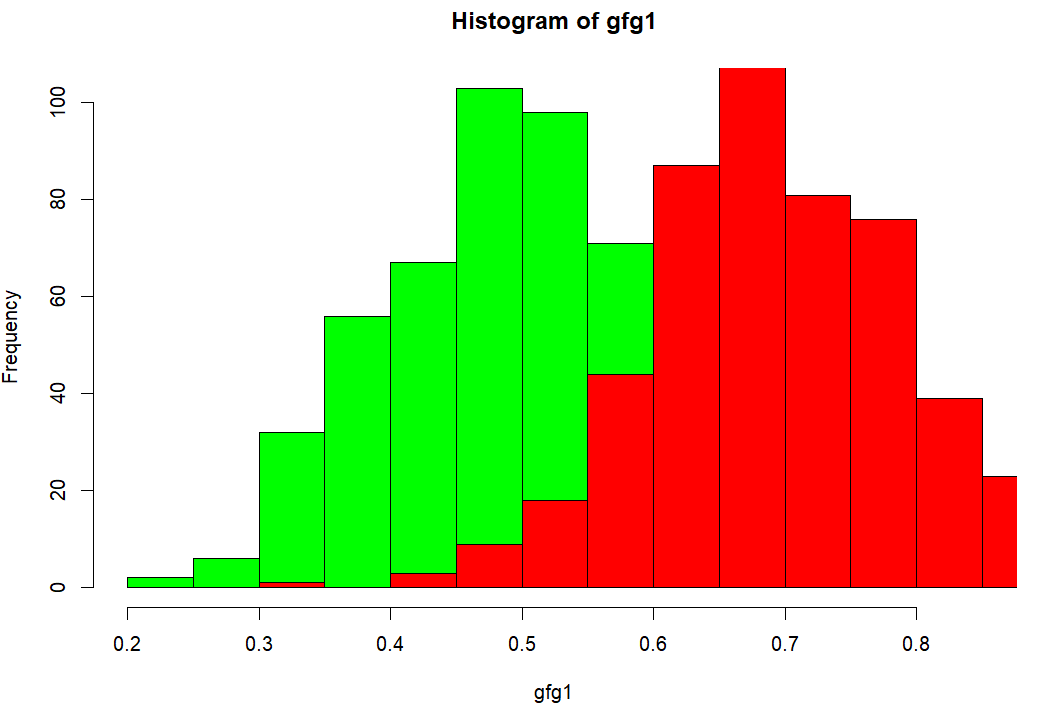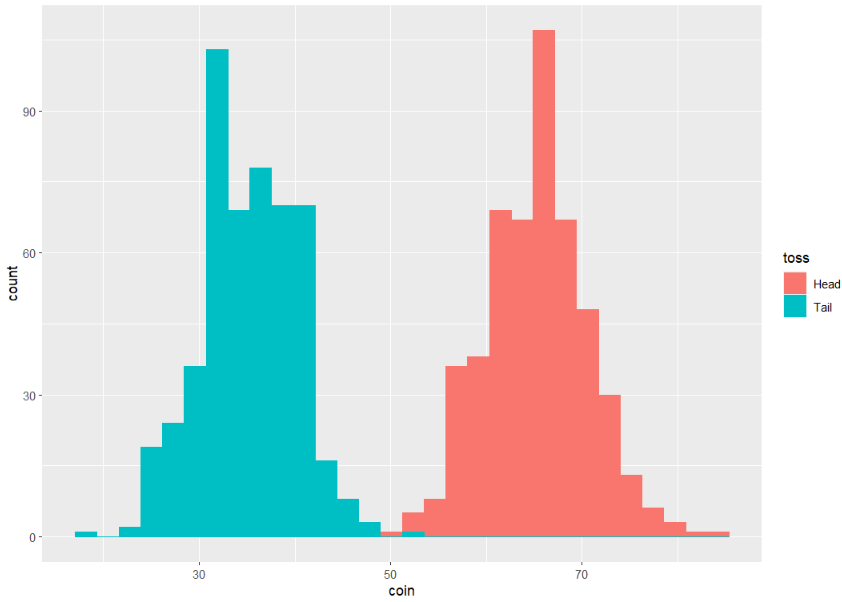# How to Create a Histogram of Two Variables in R?

• Last Updated : 19 Dec, 2021

In this article, we will discuss how to create a histogram of two variables in the R programming language.

## Method 1: Creating a histogram of two variables with base R

In this approach to create a histogram pf two variables, the user needs to call the hist() function twice as there is two number of variables, and with the second hist() function the user needs to use the special argument of this function ‘add’  with which both the histogram with different variables will be plotted on the single plot.

Syntax:

Parameters:

• x: a vector of values for which the histogram is desired.
• col: a colour to be used to fill the bars
• add: to merge the histogram

Example:

In this example, we will be creating the histogram using the hist() function with variables with random data points of 500 entries into the same plot.

## R

 `# Variable-1 with 500 random data points``gfg1<-``rnorm``(500,mean=0.5,sd=0.1) `` ` `# Variable-2 with 500 random data points``gfg2<-``rnorm``(500,mean=0.7,sd=0.1)  `` ` `# histogram of variable-1``hist``(gfg1,col=``'green'``)`` ` `# histogram of variable-2``hist``(gfg2,col=``'red'``,add=``TRUE``)`

Output:## Method 2: Creating a histogram of two variables with ggplot2 package

In this method, to create a histogram of two variables, the user has to first install and import the ggplot2 package, and then call the geom_histrogram with the specified parameters as per the requirements and needs to create the dataframe with the variable to which we need the histogram in the R programming language.

To install & import the ggplot2 package, the user needs to use the below syntax:

```Install - install.package('ggplot2')
Import - library(ggplot2)                  ```

Example:

## R

 `# load the package``library``(ggplot2)`` ` `# create a dataframe``# with mean and standard deviation``gfg < - ``data.frame``(toss=``factor``(``rep``(``c``(``"Head"``, ``"Tail"``), each=500)),``                   ``coin=``round``(``c``(``rnorm``(500, mean=65, sd=5), ``                                ``rnorm``(500, mean=35, sd=5))))`` ` `# plot the data using ggplot``ggplot``(gfg, ``aes``(x=coin, fill=toss, color=toss)) +``geom_histogram``(position=``"identity"``)`

Output:My Personal Notes arrow_drop_up